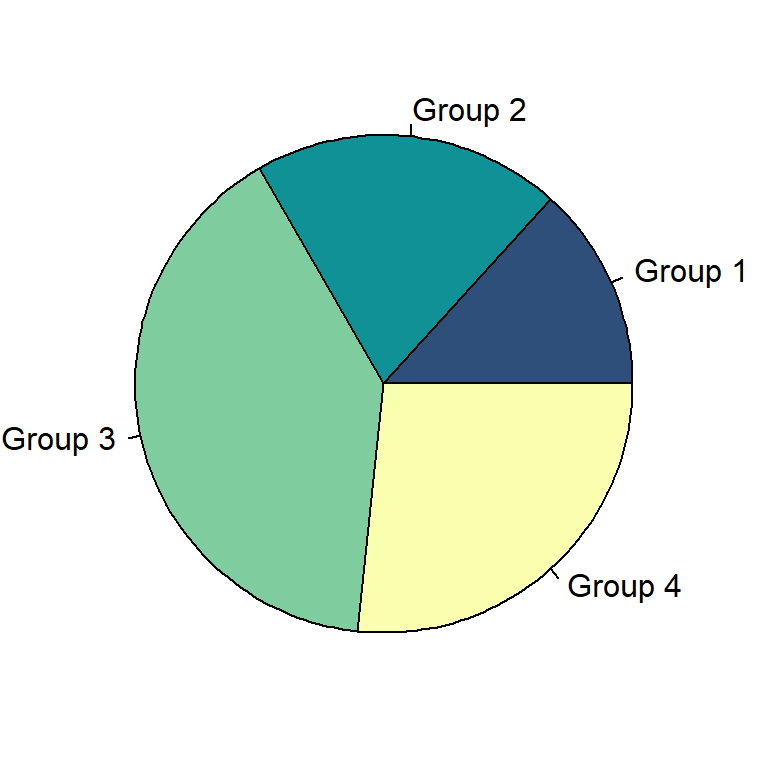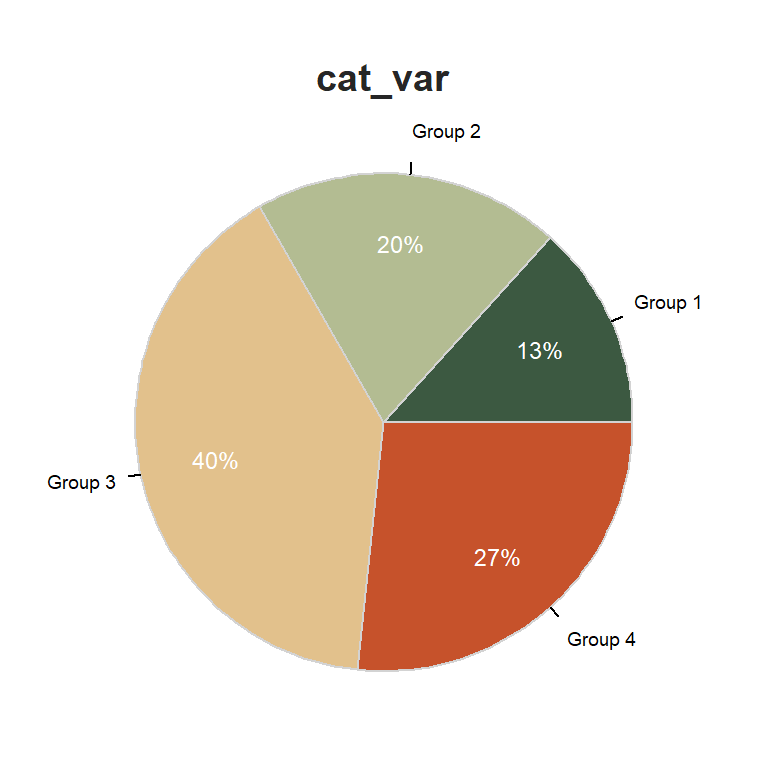# Pie chart with categorical data in R

## Pie chart with categorical data with `pie`

In order to create a pie chart in base R from a categorical variable you just need to create a table with your data with the `table` function and pass it to the `pie` function.

``````# Categorical data
cat_var <- factor(c(rep("Group 1", 10),
rep("Group 2", 15),
rep("Group 3", 30),
rep("Group 4", 20)))

# Create a table from the data
cat <- table(cat_var)

# Pie
pie(cat,
col = hcl.colors(length(cat), "BluYl"))``````## Pie chart with categorical with `PieChart`

If you want to display the group labels, in addition to the corresponding percentage for each group, you can use `PieChart` from `lessR`. This function also displays a table of frequencies and proportions and performs a Chi-square test for checking the equality of probabilities. Note that the data must be a variable of a data frame.

In addition, you can convert the pie chart into a doughnut chart if needed, increasing the value of `hole`.``````# install.packages("lessR")
library(lessR)

# Categorical data
cat_var <- factor(c(rep("Group 1", 10),
rep("Group 2", 15),
rep("Group 3", 30),
rep("Group 4", 20)))

# Store the variable as data frame
cat <- data.frame(cat_var)

# Pie
cols <-  hcl.colors(length(levels(cat_var)), "Fall")
PieChart(cat_var, data = cat, hole = 0,
fill = cols,
labels_cex = 0.6)``````